Ch 6. Entropy Multimedia Engineering Thermodynamics Entropy Tds Relations EntropyChange IsentropicProcess IsentropicEfficiency EntropyBalance (1) EntropyBalance (2) ReversibleWork
 Chapter 1. Basics 2. Pure Substances 3. First Law 4. Energy Analysis 5. Second Law 6. Entropy 7. Exergy Analysis 8. Gas Power Cyc 9. Brayton Cycle 10. Rankine Cycle Appendix Basic Math Units Thermo Tables Search eBooks Dynamics Fluids Math Mechanics Statics Thermodynamics Author(s): Meirong Huang Kurt Gramoll ©Kurt GramollTHERMODYNAMICS - THEORY Entropy Change of Pure Substance In engineering analysis, isentropic efficiency is a parameter to measure the degree of degradation of energy in steady-flow devices. It involves a comparison between the actual performance of a device and the performance that would be achieved under idealized circumstances for the same inlet and exit states. Although there exits heat transfer between the device and its surroundings, most steady-flow devices are intended to operate under adiabatic condition. Hence, normally an isentropic process is chosen to serve as the idealized process. Recall, if the inlet is denoted by subscript 1 and exit is denoted by subscript 2, the energy balance for a one-inlet-one-exit control volume is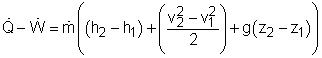Isentropic Efficiency of Turbines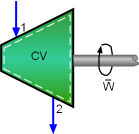Schematic of a Turbine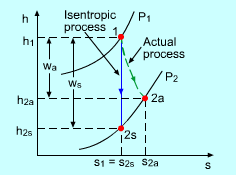h-s Diagram of the Actual and Isentropic Processes of an Adiabatic Turbine For an adiabatic turbine which undergoes a steady-flow process, its inlet and exit pressures are fixed. Hence, the idealized process for turbine is an isentropic process between the inlet and exit pressures. The desired output from a turbine is the work output. Hence, the definition of isentropic efficiency of turbine is the ratio of the actual work output of the turbine to the work output of the turbine if the turbine undergoes an isentropic process between the same inlet and exit pressures.       ηT = Actual turbine work/Isentropic turbine work             = wa/ws wa and ws can be obtained from the energy balance of the turbine. Usually the kinetic and potential energies associated with a process through a turbine is negligible compared with the enthalpy change of the process. In this case, the energy balance of the turbine is reduced to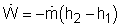The isentropic efficiency of turbine can then be written as       ηT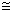(h2a - h1)/(h2s - h1) where       h1 = enthalpy at the inlet       h2a = enthalpy of actual process at the exit       h2s = enthalpy of isentropic process at the exit Isentropic Efficiency of Compressors and Pumps Compressors and pumps, when undergo a steady-flow process, consume power. The isentropic efficiency of a compressor or pump is defined as the ratio of the work input to an isentropic process, to the work input to the actual process between the same inlet and exit pressures.       ηC = Isentropic compressor (pump) work/Actual               compressor (pump) work             = ws/waSchematic of a Compressor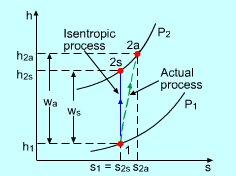h-s Diagram of the Actual and Isentropic Processes of an Adiabatic Compressor or Pump wa and ws can be obtained from the energy balance of the compressors or pumps. When the kinetic and potential energies associated with a gas flowing through a compressor are negligible compared with the enthalpy change of the gas, the energy balance of the compressor is reduced to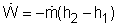The isentropic efficiency of a compressor becomes       η C(h2s - h1)/(h2a - h1) Pumps are used to handle liquid instead of gas. Since liquid is incompressible and the process is an isentropic process, the enthalpy change from inlet to exit is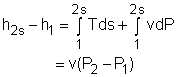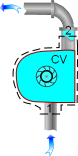Schematic of a Pump If the kinetic and potential energies are negligible, the isentropic efficiency of a pump is reduced to       η Pv(P2 - P1)/(h2a - h1) IN practice, compressors are intentionally cooled to minimize the work input. In those cases, a reversible isothermal process is served as the idealized process for compressors which are intentionally cooled, and then an isothermal efficiency is defined instead of the isentropic efficiency. The isothermal efficiency is defined as the ratio of the work input to the isothermal process, to the work input to the actual process between the same inlet and exit pressures.       ηC = Reversible isothermal compressor                work/Actual compressor work            = wt/wa Isentropic Efficiency of Nozzles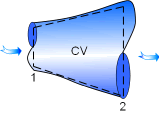Schematic of a Nozzle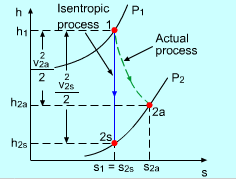h-s Diagram of the Actual and Isentropic Processes of an Adiabatic Nozzle Nozzles are devices used to accelerate the fluid velocity at the cost of pressure. The isentropic efficiency of nozzles is defined as the ratio of the actual kinetic energy at exit to the kinetic energy at the exit when the process is isentropic for the same inlet and exit pressures.       ηN = Actual KE at exit/Isentropic KE at exit             =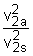There is no work interaction involved in nozzles and the potential energy change of the fluid is small. If the inlet velocity is small relatively to the exit velocity, the energy balance of a nozzle is reduced to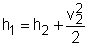Then the isentropic efficiency of nozzles becomes       ηN(h1 - h2a)/(h1 - h2s)